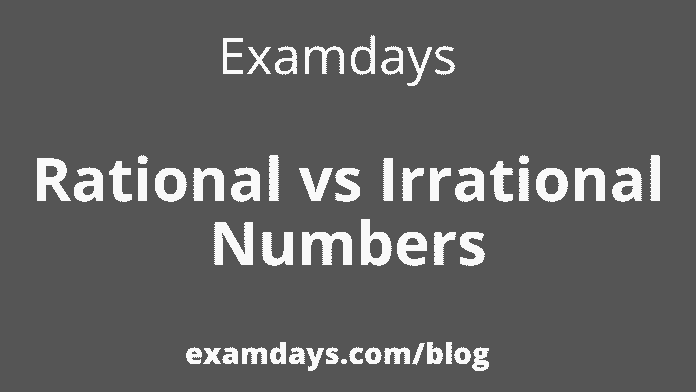# Rational vs Irrational Numbers | Difference in Numbers

0What is a rational number?: Rational numbers can be defined as numbers represented in the form p/q, where q ≠ 0. Also, any fraction that is not equal to zero is considered a rational number. So, the group of numbers that includes fractions, decimals, whole numbers, and natural numbers is rational numbers.

Five examples of rational numbers are

2, -1, 1/3, -12/23, 29/11

## Rational vs Irrational Numbers

How to identify rational numbers?

Use the following conditions to identify a rational number:

• Rational numbers are represented in the form p/q, where q≠0.
• The ratio p/q can be further simplified in normal form or decimal form
• Non-terminating decimals with repeating decimal values ​​are also considered rational numbers because they are represented in the form p/q.

What are irrational numbers?

Numbers in which they cannot be expressed as a ratio of two integers are called Irrational numbers. They are a subset of real numbers and can be expressed on a number line. And the decimal expansion of an irrational number is neither terminating nor repeating.

Irrational Numbers are non-recurring and non-repeating decimals that are part of real numbers but different from rational numbers.

2, 3, e, and Pi are some examples of irrational numbers. People have tried calculating the value of e up to a lot of decimals but still haven’t found any pattern.

Differences between Rational Numbers and Irrational Numbers

Is 0 a Rational or Irrational Number?

The number 0 is rational if it can be represented as a/b, and b is not equal to 0.

let a = 0 and b = 1 a/b = 0/1 = 0.

Since we can represent 0 as a/b, it is a rational number.

Thus, 0 is a rational number.

Is pi value a Rational or Irrational Number?

pi = 3.14159265359

Is usually taken as 22/7 but contradicting these statements

22/7 is a rational number while pi is irrational

This is just because 22/7 is taken as an approximate value of pi

Is -1 rational or irrational?

First, –1 is an integer, as 1 is an integer, and, for any number xx, xx is an integer if −x−x is also an integer.

Next, note that every integer is rational. The rational number is any number that can be expressed as the ratio of two integers, aa, and bb, where bb is nonzero. Any integer can be expressed as the ratio of itself and 1, so they are all rational.

We can conclude that –1 is a rational number for these reasons.

Conclusion:

After all the above points, it is clear that rational numbers can be expressed in fraction and decimal form. Conversely, an irrational number can only be represented in decimal form but not as a fraction. All integers are rational numbers, but non-integers are not irrational numbers.

Join Examdays Telegram

For more details about the Telegram Group, Click the Join Telegram below button.Join Membership

In case of any doubt regarding Telegram, you can mail us at [email protected].

This site uses Akismet to reduce spam. Learn how your comment data is processed.